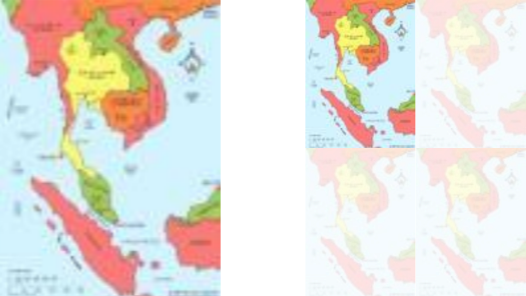#### You may also like### Building Tetrahedra

Can you make a tetrahedron whose faces all have the same perimeter?A 1 metre cube has one face on the ground and one face against a wall. A 4 metre ladder leans against the wall and just touches the cube. How high is the top of the ladder above the ground?Four rods are hinged at their ends to form a convex quadrilateral. Investigate the different shapes that the quadrilateral can take. Be patient this problem may be slow to load.

# Photocopied Map

##### Age 14 to 16 ShortChallenge Level

Using length and area scale factors
The area of the photocopied map is 25%, or a quarter, of the area of the original map.

This means that the height and width of the photocopied map are half of the height and width of the original map (see below).So the scale of the photocopied map is $\frac12$:10 000, or 1:20 000.

You could also say that, to get from the distance on the photocopied map to the distance in real life, you first multiply by 2 to find the distance on the original map, and then by 10 000 - which is the same as multiplying by 20 000.

Finding the size of 1 square kilometre on the original and photocopied maps
1:10 000 means 1 cm represents 10 000 cm, which is equal to 100 metres. So 1 kilometre would be represented by 10 cm, and so 1 square kilometre would be represented by 10 cm by 10 cm, which is 100 cm$^2$.

On the photocopy, these 100 square centimetres will become only 25 square centimetres - which is a 5 cm by 5 cm square. So now 5 cm represent 1 kilometre, and so 1 cm represents 200 m, which is 20 000 cm.

So the scale of the photocopied map is 1:20 000.

Finding the size represented by 1 square centimetre on the original and photocopied maps
1:10 000 means 1 cm represents 10 000 cm, which is equal to 100 metres. So 1 square centimetre would represent 100 m by 100 m, which is 10 000 square metres.

The area of the photocopied map is only 25% of the area of the original map, which is the same as a quarter. So 1 square centimetre on the photocopy will represent the area that 4 square centimetres represent on the original.

Each square centimetre on the original represents 10 000 square metres, so 4 cm$^2$ on the original represents 40 000 m$^2$.
So 1 cm$^2$ on the photocopy represents represents 40 000 m$^2$.

40 000 m$^2$ is 200 m by 200 m, so 1 cm on the photocopied map represents 200 m, which is 20 000 cm.

So the scale of the photocopied map is 1:20 000.

You can find more short problems, arranged by curriculum topic, in our short problems collection.